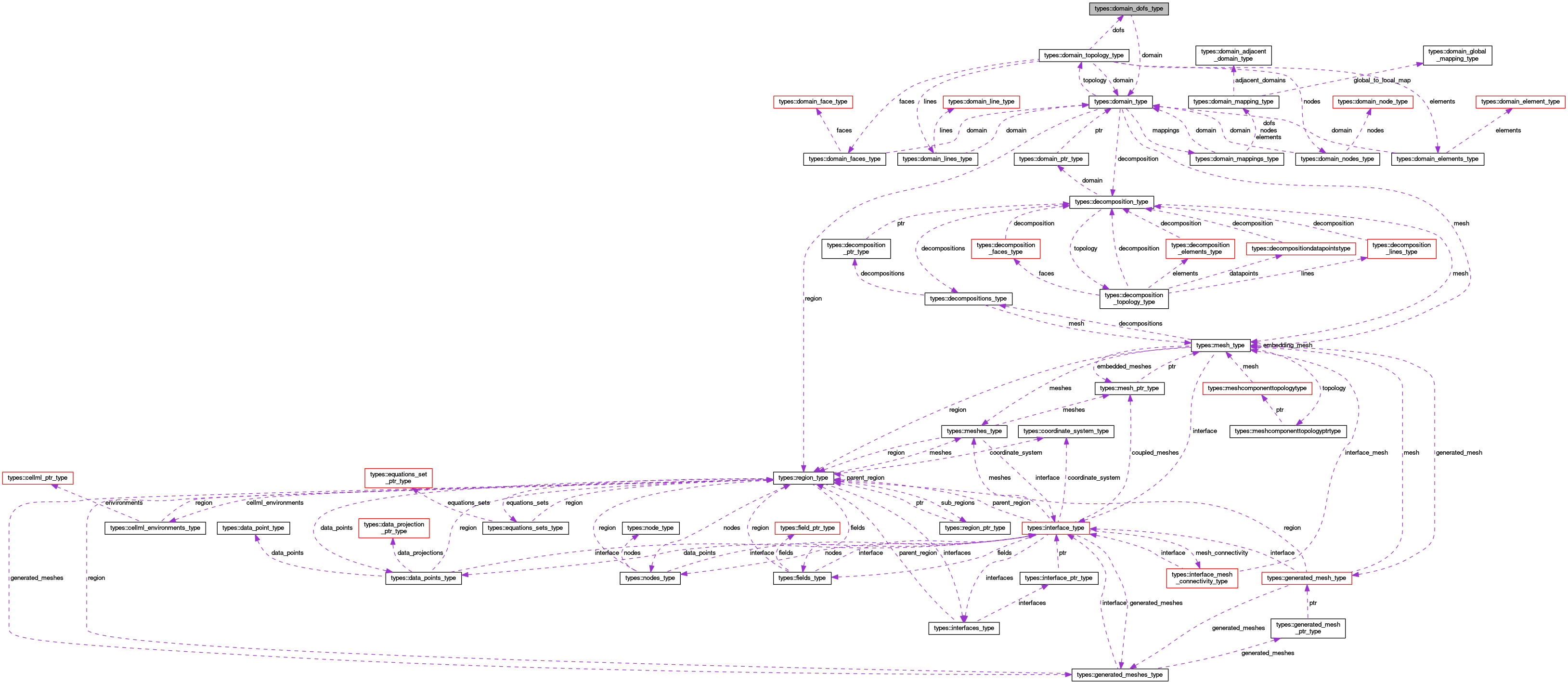OpenCMISS-Iron Internal API Documentation
types::domain_dofs_type Type Reference

Contains information on the degrees-of-freedom (dofs) for a domain. More...

Collaboration diagram for types::domain_dofs_type:[legend]

## Public Attributes

type(domain_type), pointer domain
A pointer to the domain. More...

integer(intg) number_of_dofs
The number of degrees-of-freedom (excluding ghost dofs) in the domain. More...

integer(intg) total_number_of_dofs
The total number of degrees-of-freedom (including ghost dofs) in the domain. More...

integer(intg) number_of_global_dofs
The number of global degrees-of-freedom in the domains. More...

integer(intg), dimension(:,:), allocatable dof_index
DOF_INDEX(i,ny). The index for the ny'th degree-of-freedom. When i=1 DOF_INDEX will give the global derivative version number (version_idx) associated with the dof. When i=2 DOF_INDEX will give the global derivative number (derivative_idx) associated with the dof. When i=3 DOF_INDEX will give the local node number (node_idx) associated with the dof. More...

## Detailed Description

Contains information on the degrees-of-freedom (dofs) for a domain.

Definition at line 613 of file types.f90.

## Member Data Documentation

 integer(intg), dimension(:,:), allocatable types::domain_dofs_type::dof_index

DOF_INDEX(i,ny). The index for the ny'th degree-of-freedom. When i=1 DOF_INDEX will give the global derivative version number (version_idx) associated with the dof. When i=2 DOF_INDEX will give the global derivative number (derivative_idx) associated with the dof. When i=3 DOF_INDEX will give the local node number (node_idx) associated with the dof.

Definition at line 618 of file types.f90.

 type(domain_type), pointer types::domain_dofs_type::domain

A pointer to the domain.

Definition at line 614 of file types.f90.

 integer(intg) types::domain_dofs_type::number_of_dofs

The number of degrees-of-freedom (excluding ghost dofs) in the domain.

Definition at line 615 of file types.f90.

 integer(intg) types::domain_dofs_type::number_of_global_dofs

The number of global degrees-of-freedom in the domains.

Definition at line 617 of file types.f90.

 integer(intg) types::domain_dofs_type::total_number_of_dofs

The total number of degrees-of-freedom (including ghost dofs) in the domain.

Definition at line 616 of file types.f90.